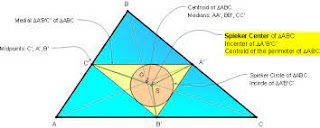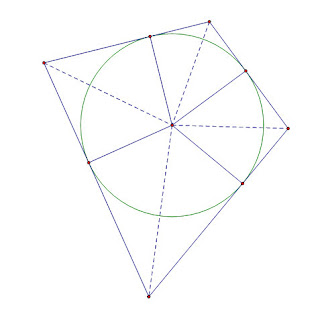## Wednesday 5 October 2022

### Centroids of Inscribable Polygons (repost)

The term centroid, or center of gravity, of a triangle usually refers to the center of gravity obtained by treating the vertices as point masses with a mass of one unit. This leads to the centroid noramally found in geometry by intersecting the three medians. If we have points (xA,yA), (xB,yB), and (xC,yC) it is well known, and easily derived, that the centroid would be the sum of the x-coordinates divided by 3, and the sum of the y-coordinates divided by three.

The centroid is defined to be the average location of all the points in the object. For a filled in triangle, this happens to be the same as the average of point masses at the vertices, but the fundamental definition is for all the points in the filled-in triangle. (Think about trying to use the vertex definitions in an ellipse.)

There are other types of centroids, and one of them has an interesting relation to the class of polygons mentioned in the title.

Another center of gravity can be found if we use the boundary of the polygon and assume it is made up of uniform rods. For a triangle, this produces a center of gravity that is at the intersection of the angle bisectors of the medial triangle (the triangle with vertices at the midpoints of the original triangle). This point is also called the Spieker Center of the triangle. To distinguish these two types of centroids, I will call the center of gravity of the vertices Gp (for point) and the center of gravity of the boundary GbSpiker Center *Wikipedia

Some interesting sidebars about the angle bisectors of the medial triangle before I continue about centers of gravity.... The angle bisectors of the medial triangle are always parallel to the angle bisector of the opposite vertex. Also, these lines are each a "cleaver" of the triangle; a term created by Dov Avishalom in a Mathematics Magazine article for a line that passes through the midpoint of one side and divides the perimeter of the triangle into two equal halves. I recently came across a paper by Tom Apostle and Mamikon A. Mnatsakanian that pointed out that in any triangle the two centroids and the incenter were always collinear. They also show that the ratio of the distances of the two centroids from the incenter is always in a ratio of 3/2. The ratio Gb/ Gp= 3/2. Then in a really nice generalization, they showed that the same property applies to any inscribable polygon, one in which each edge is tangent to a common circle, such as the quadrilateral below. They use the clever term "circumgons" in the paper for such shapes. This is really a nice term since "inscribable" and "circumscribable" are used both to describe the circle inscribed in a polygon, or the polygon circumscribed about the circle... even I get confused.

(It appears that neither of these two old papers are still active, sorry.  Here is a link to a post I wrote about cleavers and splitters ... and more)Since it is often much easier to find Gp and the incenter of inscribable polygons, this provides a clever way to find Gb. Students may be interested to know that Archimedes used the formula Area= 1/2 P r for the area of a circle, where P is the perimeter (circumference). This is, of course, simply and alternate form of the formula they learned in grade school. They may be even more surprised to find that the Archmedian formula gives the area of any inscribable polygon if P is the perimeter of the polygon, and r is the radius of the inscribed circle. The proof is very easy, and is often provided in high school textbooks for the limited case of regular polygons which would always be inscribable. The image below provides non-subtle clues to the proof.